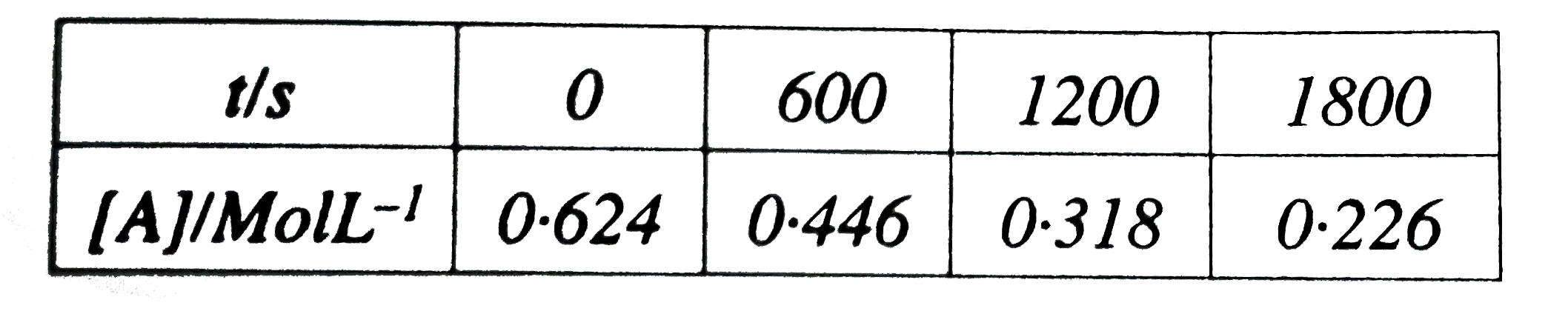# From the following data for the liequied phase reactino AtoB. determine the order of reaction and calculate its rate constant :

49 views

closed
From the following data for the liequied phase reactino AtoB. determine the order of reaction and calculate its rate constant :by (91.7k points)
selected

(i) We require to calculate the rate constant at different time intervals.
(a) k_(1)=(2*303)/(t)"log"_(10)([A_(0)])/([A_(t)])
[A_(0)]=0.624
[A_(t)]=0.446
t=600s
k_(1)=(2.303)/(600)"log" ([0.624])/([0.446])
:. k_(1)=3.838xx10^(-3)"log"[1.399]
:. k_(1)=3.838xx10^(-3)xx0.1458
:. k_(1)=5.595xx10^(-3)s^(-1)
(b) k_(2)=(2.303)/(t)"log"([A_(0)])/([A_(t)])
[A_(0)]=0624
[A_(t)]=0.318
t=1200s
k_(2)=(2.303)/(1200)"log"([0.624])/(([0.318])
:. k_(2)=1.919xx10^(-3)log [1.962]
:. k_(2)=1.919xx10^(-3) xx0.2927
:.k_(2)=5.616xx10^(-4)s^(-1)
(c) K_(3)=(2.303)/(t)"log"_(10)([A_(0)])/([A_(t)])
[A_(0)]=0.624
[A_(t)]=0.226
t=1800 s
k_(3)=(2.303)/(1200)"log"([0.624])/([0.226])
:.k_(3)=1.279xx10^(-3)log[2.7615]
:. k_(3)1.279xx10^(-3)xx0.4411
:. k_(3)=5.641xx10^(-4)s^(-1)
All the k values calculated at different time intervals are the same. This implies that the reaction obeys the intgrated rate equation of first order reaction. Hence. the reaction is a first order reaction.